# Short Introduction to BAMP

## Data example

BAMP includes a data example.

data(apc)
plot(cases[,1],type="l",ylim=range(cases), ylab="cases", xlab="year", main="cases per age group")
for (i in 2:8)lines(cases[,i], col=i)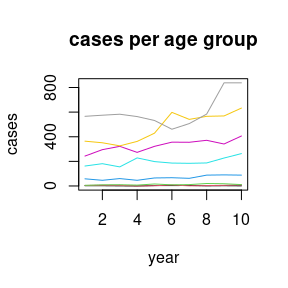For simulating APC data, see vignette(“simulation”, package=“bamp”).

## APC model with random walk first order prior

model1 <- bamp(cases, population, age="rw1", period="rw1", cohort="rw1",
periods_per_agegroup = 5)

bamp() automatically performs a check for MCMC convergence using Gelman and Rubin’s convergence diagnostic. We can manually check the convergence again:

checkConvergence(model1)
##  TRUE

Now we have a look at the model results. This includes estimates of smoothing parameters and deviance and DIC:

print(model1)
##
##  Model:
## age (rw1)  - period (rw1)  - cohort (rw1) model
## Deviance:     231.17
## pD:            36.75
## DIC:          267.91
##
##
##  Hyper parameters:                 5%           50%          95%
## age                              0.346        0.911        1.921
## period                          66.679      204.020      637.133
## cohort                          34.832       59.757       96.270
##
##
## Markov Chains convergence checked succesfully using Gelman's R (potential scale reduction factor).

We can plot the main APC effects using point-wise quantiles:

plot(model1)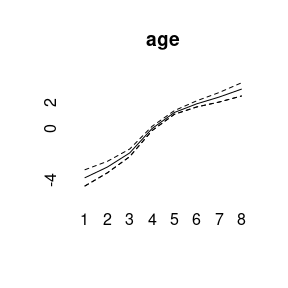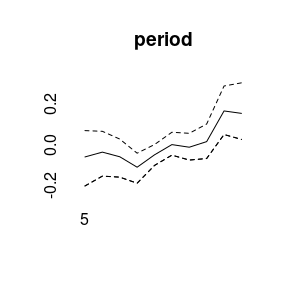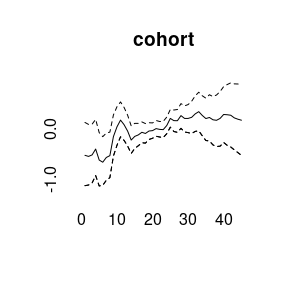More quantiles are possible:

plot(model1, quantiles = c(0.025,0.1,0.5,0.9,0.975))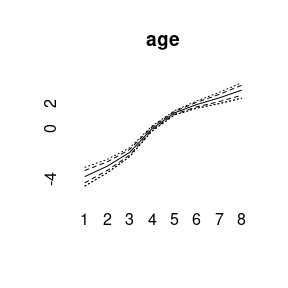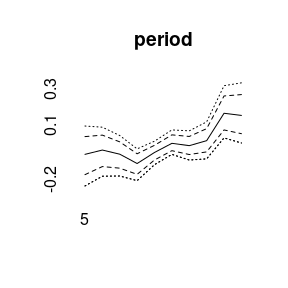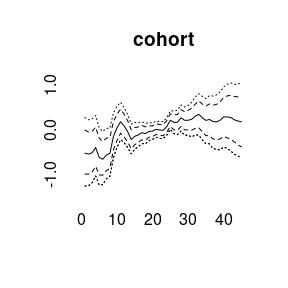For other models see vignette(“modeling”,package=“bamp”).

## Prediction

Using the prior assumption of a random walk for the period and cohort effect, one can predict cases for upcoming years.

pred <- predict_apc(object=model1, periods=3)
m<-max(pred$pr[2,,]) plot(pred$pr[2,,8],type="l", ylab="probability", xlab="year", ylim=c(0,m))
for (i in 7:1)
lines(pred\$pr[2,,i],col=8-i)
legend(1,m,col=8:1,legend=paste("Age group",1:8),lwd=2,cex=0.6)
lines(c(10.5,10.5),c(0,1),lty=2)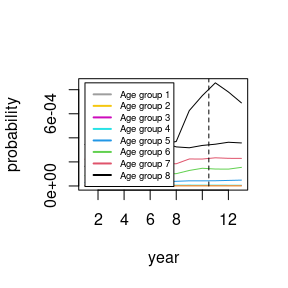More details see vignette(“prediction”,package=“bamp”).